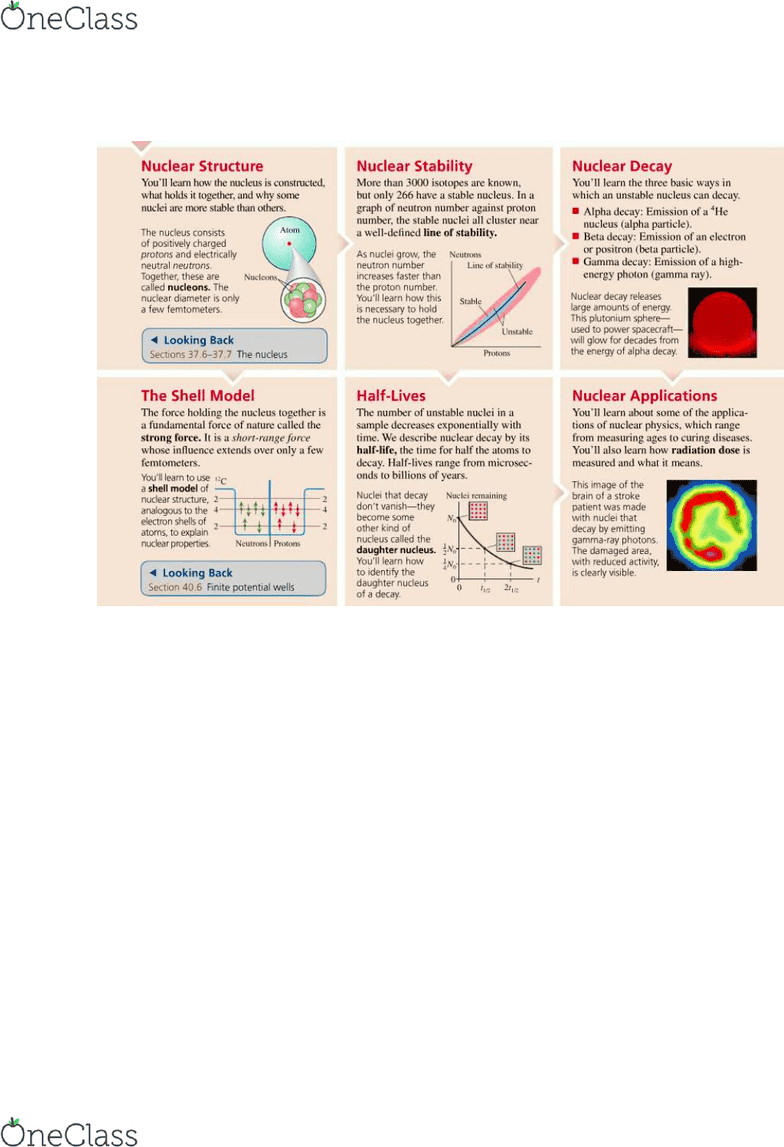Textbook Notes (280,000)
CA (170,000)
Ryerson (10,000)
PCS (40)
Chapter 42

# PCS 130 Chapter 42: Chapter 42

Department
Physics
Course Code
PCS 130
Professor
Juliana Carvalho
Chapter
42

This preview shows page 1. to view the full 5 pages of the document.Chapter 42 | Nuclear Physics
find more resources at oneclass.com
find more resources at oneclass.com

Unlock to view full version

Only page 1 are available for preview. Some parts have been intentionally blurred.42.1 Nuclear Structure
Nucleons
The nucleus is a tiny speck in the center of a vastly large atom.
Even though the nucleus is so small, it makes up 99.9% of its mass.
The nucleus is composed of protons and neutrons, together these
are called nucleons. The number of protons Z is the element’s
atomic number. In fact, an element is identified by the number of
protons in the nucleus. The mass number is represented by the
variable A and the equation is  . N is the neutron number.
Isotopes and Isobars
The atoms of an element with different values of A are called isotopes of that element. Chemical
behaviour is determined by orbiting electrons. All isotopes of one element have the same amount
of orbiting electrons and thus demonstrate the same chemical properties. Radioactive means the
nucleus is not stable. The fraction of naturally occurring nuclei represented by one particular
isotoe is called the natural abundance of that isotope. A series of nuclei having the same A
value but different values of Z and N are called isobars.
Atomic Mass
Mass of atoms is measured in atomic mass units (  ). The energy
equivalent to 1 u of mass is 931.49 MeV. Thus,  . The mass of an element on
the periodic table is the average of the isotopes of that elements mass.
Nuclear Size and Density
The radius of a nucleus with a mass number A is found to be:
  
Where    m. The volume of the nucleus is proportional to A (# of
nucleons).
1) Nucleons are incompressible. Adding more nucleons doesn’t squeeze the inner nucleons
into a smaller volume.
2) Nucleons are tightly packed.
3) Nuclear matter has a constant density.
42.2 Nuclear Stability
Binding Energy
A nucleus is a bound system. Energy is required to disperse the nucleons by
breaking the nuclear bonds between them. Binding energy is what holds the
nucleus together and is the energy you need to supply in order to disassemble
the nucleus. The binding energy of the nucleus is found as:

Where is the mass of a hydrogen atom and is the mass of a neutron. The nuclear binding
energy increases as A increases simply because there are more nuclear bonds. The binding
energy per nucleon is found easily by using this equation: .
find more resources at oneclass.com
find more resources at oneclass.com